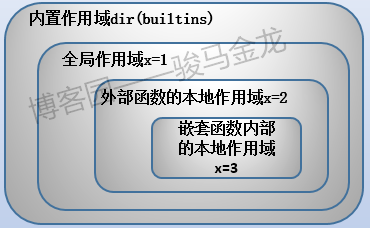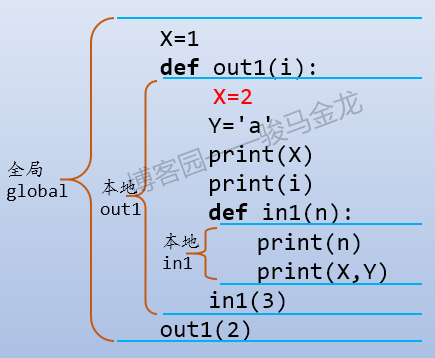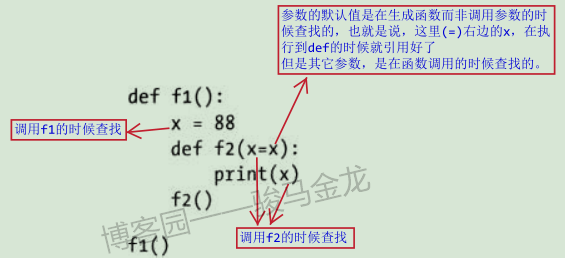# Python作用域详述

python的变量作用域的规则非常简单，可以说是所有语言中最直观、最容易理解的作用域。

x=1
def f():
x=3
g()
print("f:",x)   # 3

def g():
print("g:",x)   # 1

f()
print("main:",x)    # 1


## python作用域规则简介• 内置作用域是预先定义好的，在__builtins__模块中。这些名称主要是一些关键字，例如open、range、quit等
• 全局作用域是文件级别的，或者说是模块级别的，每个py文件中处于顶层的变量都是全局作用域范围内的变量
• 本地作用域是函数内部属于本函数的作用范围，因为函数可以嵌套函数，嵌套的内层函数有自身的内层范围
• 嵌套函数的本地作用域是属于内层函数的范围，不属于外层

X=1
def out1(i):
X=2
Y='a'
print(X)
print(i)
def in1(n):
print(n)
print(X,Y)
in1(3)
out1(2)## 内置作用域

>>> import builtins
>>> dir(builtins)
['ArithmeticError', 'AssertionError', 'AttributeError', 'BaseException', 'BlockingIOError', 'BrokenPipeError', 'BufferError', 'BytesWarning', ...............
'range', 'repr', 'reversed', 'round', 'set', 'setattr', 'slice', 'sorted', 'staticmethod', 'str', 'sum', 'super', 'tuple', 'type', 'vars', 'zip']


## 变量掩盖和修改规则

x=3
def g():
print(x)  # 引用全局变量x


x=3
def g():
x=2   # 定义并赋值本地变量x
print(x)  # 引用本地变量x


python是一种解释性语言，读一行解释一行，读了下一行就忘记前一行(详细见下文)。所以在使用变量之前必须先进行变量的定义(声明)

def g():
print(x)
x=3
g()


UnboundLocalError: local variable 'x' referenced
before assignment


x=1
def g():
print(x)
x=2
g()


x=1
def g():
x=2
print(x)
g()


g()
def g():
x=2
print(x)


NameError: name 'g' is not defined


x=1
def f():
x=3
g()
print("f:",x)   # 3

def g():
print("g:",x)   # 1

f()
print("main:",x)    # 1


x=1
def f():
x=3
g()
print("f:",x)

f()   # 报错

def g():
print("g:",x)


x=3

def f1():
x += 3
print(x)
f1()


UnboundLocalError: local variable 'x' referenced before assignment


## 关于全局变量

• 每个py文件(模块)都有一个自己的全局范围
• 文件内部顶层的，不在def区块内部的变量，都是全局变量
• def内部声明(赋值)的变量默认是本地变量，要想让其变成全局变量，需要使用global关键字声明
• def内部如果没有声明(赋值)某变量，则引用的这个变量是全局变量

x=2
def f():
print(x)
f()             # 输出2


x=2
def f():
x=3
print(x)
f()        # 输出3
print(x)   # 输出2


### global关键字

x=2
def f():
global x
x=3
print(x)

f()          # 输出3
print(x)     # 输出3


global可以声明一个或多个变量为全局变量，多个变量使用逗号隔开，也可以声明事先不存在的变量为全局变量：

x=2
def f():
global x,y
x,y = 3,4
print(x,y)
f()
print(x,y)


global x=2


x=2
def f():
global x,y
print(y)


NameError: name 'y' is not defined


x=2
def f():
global x,y
y=3
print(y)


global修饰的变量必须在它的赋值之前，所以下面的是错的，因为y=2首先将它声明为本地变量了。

def f():
y=2
global y


### 全局变量的不安全性

x=2
def f():
global x
x=3

def g():
global x
x=4

f()或g()
print(x)


### 访问其它模块中的全局变量

python中一个文件一个模块，在模块1中可以导入模块2中的属性(例如全局变量)。

x=3


a.py文件中：

import b
print(b.x)
b.x=4


b.py文件中：

x=3
def setx(n)
global x
x=n


a.py文件中：

import b
b.setx(54)   # 将b.x变量设置为54


### 其它访问全局变量的方法

x=3

def f():
global x
x += 2

def f1():
x=4        # 本地变量

def f2():
x=4             # 本地变量
import b
b.x += 2  # 全局变量

def f3():
x=4            # 本地变量
import sys
glob = sys.modules['b']
glob.x += 2    # 全局变量

def test():
print("aaa",x)            # 输出3
f();f1();f2();f3()
print("bbb",x)            # 输出9


import b
b.test()


## nonlocal关键字

• (1).内层函数中赋值的变量是属于内层、不属于外层的本地变量
• (2).内层函数中使用的未在当前内层函数中赋值的变量是属于外层、全局的变量

x=3

def f1():
x=4
def f2():
y=5
print(x,y)
f2()
f1()


nonlocal语句可以修饰内层函数中的变量使其成为它上一层函数的变量。它的用法和global基本相同，修饰多个变量的时候，需要逗号隔开。但和global有一点不同，global修饰的变量可能事先并未存在于全局作用域内，但nonlocal修饰的变量必须已经存在于上层或上上层(或更多层)函数，不能只存在于全局(见下面示例)。

x=3

def f1():
x=4          # f1的本地变量
def f2():
x=5      # f2的本地变量
def f3():
nonlocal x  # f2的本地变量
print("f3:",x)  # 输出5
x=6
f3()
print("f2:",x)  # 被修改，输出6
f2()
f1()


f3: 5
f2: 6


x=3

def f1():
x=4
def f2():
def f3():
nonlocal x      # f1()的本地
print("f3:",x)  # 输出4
x=6             # 修改f1()的本地
f3()
print("f2:",x)   # 输出6
f2()
print("f1:",x)       # 输出6
f1()


x=3
def f1():
x=4
def f2():
print(x)
x=3
f2()
f1()


x=3
def f1():
x=4
def f2():
x += 3
print(x)
f2()
f1()


UnboundLocalError: local variable 'x' referenced before assignment


### 访问外层函数变量的其它方法

x=3
def f1():
x=4
def f2(x=x):
x += 3
print("f2:",x)
x=5
f2()
print("f1:",x)
f1()


f2: 7
f1: 5### 避免函数嵌套

def f1():
x=3
def f2():
nonlocal x
print(x)
f2()
f1()


def f1():
x=3
f2(x)

def f2(x):
print(x)

f1()


## 循环内部的函数

def f1():
list1 = []
for i in range(5):
def n(x):
return i+x
list1.append(n)
return list1

mylist = f1()
for i in mylist: print(i)
print(mylist(2))
print(mylist(2))


<function f1.<locals>.n at 0x02F93660>
<function f1.<locals>.n at 0x02F934B0>
<function f1.<locals>.n at 0x02F936A8>
<function f1.<locals>.n at 0x02F93738>
<function f1.<locals>.n at 0x02F93780>
6
6


(注：对于此现象，各语言基本都是如此，本节稍作解释，真正的本质原因在本文的最后一节做了额外的补充解释代码块细述)。

def f1():
for i in range(5):
def n():
print(i)
return n

f1()()


x=3
def f():
print(x)


def f1():
list1 = []
for i in range(5):
def n(x,i=i):
return i+x
list1.append(n)
return list1


## 再述作用域规则

python的作用域是词法作用域，这意味着函数的定义位置决定了它所看见的变量。除了词法作用域，还有动态作用域，动态作用域意味着函数的调用位置决定了它所看见的变量。关于词法、动态作用域，本文不多做解释，想要了解的话，可以参考一文搞懂：词法作用域、动态作用域、回调函数、闭包

x=1
def f():
x=3
g()
print("f:",x)   # 3

def g():
print("g:",x)   # 1

f()
print("main:",x)    # 1


1. 调用函数之前，理论上要先定义好函数，但这里g()的调用似乎看上去比g()的定义更先
2. f()中调用g()时，为什么g()输出的是1而不是3

• (1).首先声明def f()，在此期间会创建一个本地变量x，并且print("f:",x)中的x指向这个本地变量；
• (2).然后声明g()，在此期间，g()的定义语句不在f()内部，而是在全局范围，所以它看见的是x是全局x，所以print("g:",x)中的x指向全局变量x；

x=3

def f1():
x=4
def f2():
print(x)
x=5
f2()
f1()      # 输出5


## 代码块细述(必看)

A Python program is constructed from code blocks. A block is a piece of Python program text that is executed as a unit. The following are blocks: a module, a function body, and a class definition. Each command typed interactively is a block. A script file (a file given as standard input to the interpreter or specified as a command line argument to the interpreter) is a code block. A script command (a command specified on the interpreter command line with the ‘-c’ option) is a code block. The string argument passed to the built-in functions eval() and exec() is a code block.


1. 模块文件是一个代码块
2. 函数体是一个代码块
3. class的定义是一个代码块
4. 交互式(python idle)的每一个命令行都是一个独立的代码块
5. 脚本文件是一个代码块
6. 脚本命令是一个代码块(python -c "xxx")
7. eval()和exec()中的内容也都有各自的代码块

>>> x=2000
>>> y=2000
>>> x is y
False
>>> x=2000;y=2000
>>> x is y
True


>>> x=2000;y=2000
>>> x is y
True


idle工具中每个命令都是独立的代码块，但是py文件却是一个完整的代码块，其内还可以嵌套其它代码块(如函数、exec()等)。所以，如果上面的分行赋值语句放在py文件中，得到的结果将是True。

x = 2000
y = 2000
print(x is y)   # True
def f1():
z=2000
z1=2000
print(x is z)   # False
print(z is z1)  # True

f1()


python先读取x=2000，并在内存中创建一个属于全局作用域的2000数据对象，再解释y=2000的时候，发现这个全局对象2000已经存在了(因为x和y同处于全局代码块内)，所以不会再额外创建新的2000对象。这里反映出来的结果是"同一个代码块内，虽然仍然是读一行解释一行，但在退出这个代码块之前，不会忘记这个代码块中的内容，而且会统筹安排这个代码块"。

x = 3
def f1():
print(x)
x=4
f1()


UnboundLocalError: local variable 'x' referenced before assignment


>>> x=256
>>> y=256
>>> x is y
True


x = 3
def f1():
y=3
print(x is y)   # True

f1()


def f1():
for i in range(5):
def n():
print(i)
return n

f1()()


python首先解释def f1()的代码块，会记录属于这个代码块作用域内的变量i和n，但i和n都不会赋值，也就是说暂时并不知道变量n是一个函数变量。

def f1():
for i in range(5):
def n():
print(i)
n()
return n

f1()


0
1
2
3
4


def f1():
L = []
for i in range(5):
def n(i=i):
print(i)
L.append(n)
return L

f1()()
f1()()
f1()()
f1()()
f1()()


0
1
2
3
4


Linux运维交流群：921383787

posted @ 2018-11-07 19:11  骏马金龙  阅读(2368)  评论(1编辑  收藏  举报